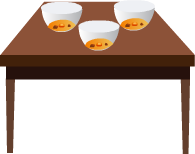# How to Multiply Fractions?

Print
Rate 0 stars
Common Core
• Lesson size:
Message preview:
Someone you know has shared lesson with you:

To play this lesson, click on the link below:

https://www.turtlediary.com/lesson/multiplying-fractions.html

Hope you have a good experience with this site and recommend to your friends too.

Login to rate activities and track progress.
Login to rate activities and track progress.

Multiplying fractions with whole numbers is much easier than adding fractions.

Let us learn this by following examples :

## Example 1

If we have four children and each has two cookies, how many cookies are there in all?If we multiply 4 x 2, we find that there are 8 cookies total.

But what if we have four children and each has
2
3

Just like with whole numbers, we can use multiplication to solve a problem like this.

Multiply the numerator by the whole number, and leave the denominator the same.

We need to multiply 4 x
2
3
First, multiply the numerator by the whole number.

4 x 2 = 8

Remember, the denominator stays the same, so our answer is:

8
3

Since the numerator is larger than the denominator, you can tell that this fraction is bigger than one.

You can restate it as 2
2
3
.

Here is how we did it. To change an improper fraction to a mixed number, you must divide the numerator by the denominator. Then, the mixed number takes the following form :

• The answer becomes the whole number
• The remainder becomes the numerator
• The denominator stays the same## Example 2

There are three bowls of soup on a table. Each bowl is
1
5
full. How much soup is there in total?We need to multiply 3 x
1
5
.

3 x 1
5
=
3
5

There is
3
5
of a bowl soup in total.

## How to Multiply Fractions?

• Fractions represent parts of whole numbers
• Numerator (number on top) represents how many parts of the whole you have
• Denominator (number on the bottom) represents how many equal parts the whole is divided into.
• Multiplying Fractions by a Whole Number: Multiply the numerator by the whole number, and leave the denominator the same.

## Similar Lessons

Become premium member to get unlimited access.